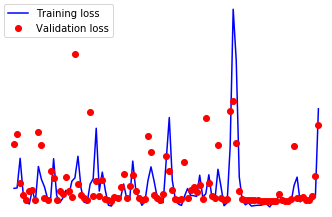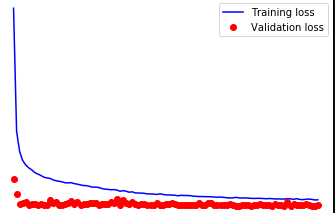# Validation Loss not Decreasing for Autoencoder

Finally got fed up with tensorflow and am in the process of piping a project over to pytorch. So far I’ve found pytorch to be different but MUCH more intuitive.

One of my nets is a good old fashioned autoencoder I use for anomaly detection of unlabelled data. I’ve set it up to periodically report my current training and validation loss and have come across a head scratcher. My training loss improves about what I’d expect (although faster would be great), but my validation loss remains essentially the same. I’ve perused the forums here and can’t find anything that helps. Admittedly, while I can build nets pretty well in tensorflow, this could just be a stupid error on my part. I know it isn’t the data as the same data, formatted exactly the same way performs well in tensorflow. And I have… a lot of data. So no issues there. I’ll post a sample below of the minimum working code that reproduces the error.

If you want machine specs, I’d be happy to post if they’re relevant (three gpus).

Thanks!

Formatting data and defining the autoencoder

``````train_data = torch.from_numpy(df[fullData].values[:train])
val_data = torch.from_numpy(df[fullData].values[validate:])

batchsize = 1024

class Model(nn.Module):

def __init__(self, input_size, output_size, droprate):
super(Model, self).__init__()
self.en1 = nn.Linear(input_size, 640)
self.dp1 = nn.Dropout(droprate)
self.en2 = nn.Linear(640, 320)
self.dp2 = nn.Dropout(droprate)
self.en3 = nn.Linear(320, 160)
self.dp3 = nn.Dropout(droprate)
self.en4 = nn.Linear(160, 80)
self.dp4 = nn.Dropout(droprate)
self.dec1 = nn.Linear(80, 160)
self.dp5 = nn.Dropout(droprate)
self.dec2 = nn.Linear(160, 320)
self.dp6 = nn.Dropout(droprate)
self.dec3 = nn.Linear(320, 640)
self.dp7 = nn.Dropout(droprate)
self.dec4 = nn.Linear(640, output_size)

def forward(self, ins):
x = F.elu(self.en1(ins))
x = self.dp1(x)
x = F.elu(self.en2(x))
x = self.dp2(x)
x = F.elu(self.en3(x))
x = self.dp3(x)
x = F.elu(self.en4(x))
x = self.dp4(x)

x = F.elu(self.dec1(x))
x = self.dp5(x)
x = F.elu(self.dec2(x))
x = self.dp6(x)
x = F.elu(self.dec3(x))
x = self.dp7(x)
output = F.elu(self.dec4(x))

return output
``````

Aaaaand doing the training

``````model = Model(input_size, output_size, 0.5)
if torch.cuda.device_count() > 1:
model = nn.DataParallel(model)
model.to(device)

num_epochs = 10
iters = 0
model = model.double()
validate = 100
best_val_loss = 100
vals = []
losses = []
for epoch in range(num_epochs):
model.train()

iters += 1
inputs = batch.to(device)
output = model(inputs)
train_loss = criterion(output, inputs)

train_loss.backward()
optimizer.step()

if iters % validate == 0:
val_loss = 0
model.eval()

for val in (val_iter):
val = val.to(device)

vals.append(val_loss.item())
iterations.append(iters)
losses.append(train_loss.item())
``````

Each time `iters % validate == 0`, you append only the last `train_loss` to `lossess`, and the last `val_loss` to `vals`.
Maybe you’d want to accumulate them, and append their means for example.

I went ahead and modified my code to accumulate the averages of the values as you suggested. My results look a little better but I think maybe more so just confirms the problem further haha

For reference my:
starting training loss was 0.016 and validation was 0.0019,
final training loss was 0.004 and validation loss was 0.0007.

And here’s a viz of the losses over ten epochs of training. Based on this, I think the model is improving and I’m not calculating validation loss correctly, but I can’t figure out anything I’m doing wrong!What is curious is that the validation loss seems to converge 10 times faster than the training loss.
To better understand what is going on, could you:

• Do validation at a higher frequency ; for example at `validate = 20`
• Try to train it without dropout… Just to see if something changes

By the way, a `nn.Dropout()` layer has no parameters. So you can define a single layer instead of seven : `self.dp = nn.Dropout(droprate)`
And in forward call this layer multiple times : `x = self.dp(x)`.

It’s funny that you mention that about the dropout because right before reading you comment I had a little facepalm moment when I realized just that.

I also tried taking out the dropout and what happened was the validation accuracy had the same behavior (where it was essentially the same over time), but the accuracy improved to the range of 1e-5.

When I validate more often (which was a good suggestion) I think it’s uncovered a little more granular view of it not improving haha.

This is validating every 20 without dropout for 10 epochsThis is the same, but with dropout rate of 0.5 between each layerWhat I might need to do is just copy some other project and try and run it and see if I can replicate their results. The validation scores are good, my worry is just that since they aren’t improving, 1) something is wrong, and 2) that limits how accurate my model can be in the long run

Yeah, not sure why, but pirating this code got me the kind of performance one would expect from a neural net. I’ll have to look into the differences a little more to understand why it’s working while my model doesn’t.